# E-function

(diff) ← Older revision | Latest revision (diff) | Newer revision → (diff)

The concept of-functions was introduced by C.L. Siegel in [a1], p. 223, in his work on generalizations of the Lindemann–Weierstrass theorem.

Consider a Taylor series of the form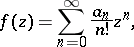where the numbers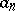belong to a fixed algebraic number field (cf. also Algebraic number; Field)(). Suppose it satisfies the following conditions:

i)satisfies a linear differential equation with polynomial coefficients;

ii) for anyone has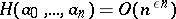. Thenis called an-function. Here, the notation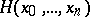stands for the so-called projective height, given by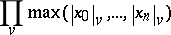for any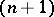-tuple. The product is taken over all valuations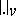of(cf. also Norm on a field). When theare rational numbers,is simply the maximum of the absolute values of thetimes their common denominator. As suggested by their name,-functions are a variation on. A large class of examples is given by the hypergeometric functions (cf. Hypergeometric function) of the form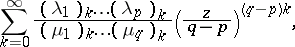where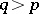,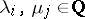for all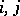and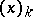is the Pochhammer symbol, given by. Motivated by the success of the Lindemann–Weierstrass theorem and techniques of A. Thue and W. Maier, Siegel was the first to define and study them. He found a number of transcendence results on values of-functions at algebraic points. These results were published in 1929 and later, in 1949, a more systematic account appeared in [a2]. Unfortunately, Siegel's main result contains a normality condition on the differential equations which, in practice, seemed very hard to verify. This condition was removed by A.B. Shidlovskii, around 1955 [a3]. Roughly speaking, ifare-functions that are algebraically independent over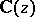(cf. Algebraic independence), then the valuesare algebraically independent overfor all algebraicexcepting a known finite set. Thus, proving the algebraic independence of values of-functions at algebraic points has been reduced to the problem of showing algebraic independence overof functions satisfying linear differential equations. During the last thirty years the latter problem has been the object of study of a school of Russian mathematicians and a few non-Russian mathematicians as well. Many of these results are contained in [a4]. In recent years, F. Beukers, W.D. Brownawell and G. Heckman studied these problems with the powerful techniques from differential Galois theory, see [a5], [a6], [a7], and also Galois differential group.

How to Cite This Entry:
E-function. Encyclopedia of Mathematics. URL: http://encyclopediaofmath.org/index.php?title=E-function&oldid=14699
This article was adapted from an original article by F. Beukers (originator), which appeared in Encyclopedia of Mathematics - ISBN 1402006098. See original article